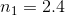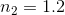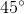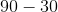GRE Subject Test: Physics : Reflection and Refraction

Example Questions

Example Question #1 : Reflection And Refraction

A beam of light travels through a medium with index of refractionuntil it reaches an interface with another material, with index of refraction. No light is transmitted into the second material. At what angle (measured from the plane of the interface between the two materials) does the beam hit the second material?This situation is impossible.Explanation:

Total internal reflection occurs at the angle:However, this angle is measured from a line normal to the plane of the interface; the angle we want, therefore, is, which is.

All GRE Subject Test: Physics Resources